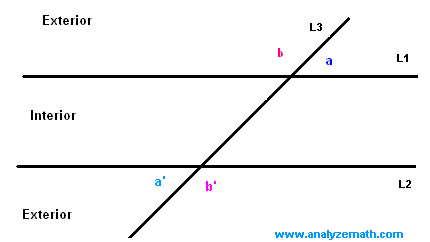# Parallel Lines and Angles

Tutorial on angles formed when a transversal L3 intersects two parallel lines L1 and L2. You may also want to solve problems related to Parallel Lines and Angles.

## Corresponding Angles

All corresponding angles are congruent and are equal in measure. The pairs of angles a and a ' , b and b ' , c and c ' and d and d ' are corresponding angles.(see figure below).
measure of angle a = measure of angle a '
measure of angle b = measure of angle b '
measure of angle c = measure of angle c '
measure of angle d = measure of angle d '## Alternate Interior Angles

The pairs of alternate interior angles in the figure below are d and d ' and c and c '. The alternate interior angles are congruent and equal in measure. (see figure below)
measure of angle d = measure of angle d '
measure of angle c = measure of angle c '## Alternate Exterior Angles

The pairs of alternate exterior angles in the figure below are a and a ' and b and b '. The alternate exterior angles are congruent and equal in measure. See figure below)
measure of angle a = measure of angle a '
measure of angle b = measure of angle b '## More References and Links to Geometry

Geometry Tutorials, Problems and Interactive Applets.